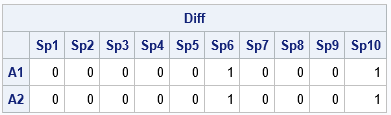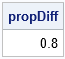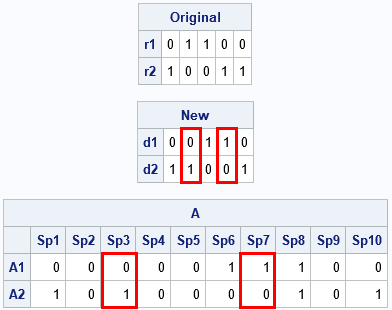Binary matrices are used for many purposes. I have previously written about how to use binary matrices to visualize missing values in a data matrix. They are also used to indicate the co-occurrence of two events. In ecology, binary matrices are used to indicate which species of an animal are present in which ecological site. For example, if you remember your study of Darwin's finches in high school biology class, you might recall that certain finches (species) are present or absent on various Galapagos islands (sites). You can use a binary matrix to indicate which finches are present on which islands.

Recently I was involved in a project that required performing a permutation test on rows of a binary matrix. As part of the project, I had to solve three smaller problems involving rows of a binary matrix:

1. Given two rows, find the locations at which the rows differ.
2. Given two binary matrices, determine how similar the matrices are by computing the proportion of elements that are the same.
3. Given two rows, swap some of the elements that differ.

This article shows how to solve each problem by using the SAS/IML matrix language. A future article will discuss permutation tests for binary matrices. For clarity, I introduce the following macro that uses a temporary variable to swap two sets of values:

```/* swap values of A and B. You can use this macro in the DATA step or in the SAS/IML language */ %macro SWAP(A, B, TMP=_tmp); &TMP = &A; &A = &B; &B = &TMP; %mend;```

### Where do binary matrices differ?

The SAS/IML matrix language enables you to treat matrices as high-level objects. You often can answer questions about matrices without writing loops that iterate over the elements of the matrices. For example, if you have two matrices of the same dimensions, you can determine the cells at which the matrices are unequal by using the "not equal" (^=) operator. The following SAS/IML statements define two 2 x 10 matrices and use the "not equal" operator to find the cells that are different:

```proc iml; A = {0 0 1 0 0 1 0 1 0 0 , 1 0 0 0 0 0 1 1 0 1 }; B = {0 0 1 0 0 0 0 1 0 1 , 1 0 0 0 0 1 1 1 0 0 }; colLabel = "Sp1":("Sp"+strip(char(ncol(A)))); rowLabel = "A1":("A"+strip(char(nrow(A))));   /* 1. Find locations where binary matrices differ */ Diff = (A^=B); print Diff[c=colLabel r=rowLabel];```The matrices A and B are similar. They both have three 1s in the first row and fours 1s in the second row. However, the output shows that the matrices are different for the four elements in the sixth and tenth columns. Although I used entire matrices for this example, the same operations work on row vectors.

### The proportion of elements in common

You can use various statistics to measure the similarity between the A and B matrices. A simple statistic is the proportion of elements that are in common. These matrices have 20 elements, and 16/20 = 0.8 are common to both matrices. You can compute the proportion in common by using the express (A=B)[:], or you can use the following statements if you have previously computed the Diff matrix:

```/* 2. the proportion of elements in B that are the same as in A */ propDiff = 1 - Diff[:]; print propDiff;```As a reminder, the mean subscript reduction operator ([:]) computes the mean value of the elements of the matrix. For a binary matrix, the mean value is the proportion of ones.

### Swap elements of rows

The first two tasks were easy. A more complicated task is swapping values that differ between rows. The swapping operation is not difficult, but it requires finding the k locations where the rows differ and then swapping all or some of those values. In a permutation test, the number of elements that you swap is a random integer between 1 and k, but for simplicity, this example uses the SWAP macro to swap two cells that differ. For clarity, the following example uses temporary variables such as x1, x2, d1, and d2 to swap elements in the matrix A:

```/* specify the rows whose value you want to swap */ i1 = 1; /* index of first row to compare and swap */ i2 = 2; /* index of second row to compare and swap */   /* For clarity, use temp vars x1 & x2 instead of A[i1, ] and A[i2, ] */ x1 = A[ i1, ]; /* get one row */ x2 = A[ i2, ]; /* get another row */ idx = loc( x1^=x2 ); /* find the locations where rows differ */ if ncol(idx) > 0 then do; /* do the rows differ? */ d1 = x1[ ,idx]; /* values at the locations that differ */ d2 = x2[ ,idx]; print (d1//d2)[r={'r1' 'r2'} L='Original'];   /* For a permutation test, choose a random number of locations to swap */ /* numSwaps = randfun(1, "Integer", 1, n); idxSwap = sample(1:ncol(idx), numSwaps, "NoReplace"); */ idxSwap = {2 4}; /* for now, hard-code locations to swap */ %SWAP(d1[idxSwap], d2[idxSwap]); print (d1//d2)[r={'d1' 'd2'} L='New']; /* update matrix */ A[ i1, idx] = d1; A[ i2, idx] = d2; end; print A[c=colLabel r=rowLabel];```The vectors x1 and x2 are the rows of A to compare. The vectors d1 and d2 are the subvectors that contain only the elements of x1 and x2 that differ. The example swaps the second and fourth columns of d1 and d2. The new values are then inserted back into the matrix A. You can compare the final matrix to the original to see that the process swapped two elements in each of two rows.

Although the examples are for binary matrices, these techniques work for any numerical matrices.

ShareDistinguished Researcher in Computational Statistics

Rick Wicklin, PhD, is a distinguished researcher in computational statistics at SAS and is a principal developer of SAS/IML software. His areas of expertise include computational statistics, simulation, statistical graphics, and modern methods in statistical data analysis. Rick is author of the books Statistical Programming with SAS/IML Software and Simulating Data with SAS.

### 1 Comment

1.Rick,
If it was just a binary matrix, I think using NOT operator would be simpler.

proc iml;
A = {0 0 1 0 0 1 0 1 0 0 ,
1 0 0 0 0 0 1 1 0 1 };
idx_diff=loc(A[+,]=1);

idx_swap = {2 4};
idx=idx_diff[idx_swap];
A[,idx]=^A[,idx];
print A;
quit;